# (SOLVED) Financial Reporting and Analysis Chapter 6, Problem 11P

Discipline: Finance

Paper Format: APA

Pages: 1 Words: 600

Question

A partial balance sheet and income statement for King Corporation follow: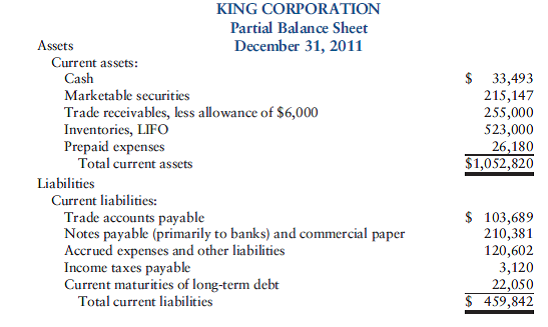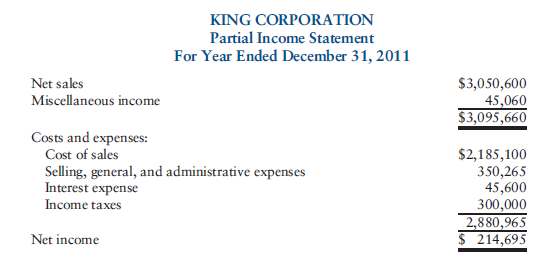Note: The trade receivables at December 31, 2010, were \$280,000, net of an allowance of \$8,000, for a gross receivables figure of \$288,000. The inventory at December 31, 2010, was \$565,000.

Required Compute the following:

a. Working capital

b. Current ratio

c. Acid-test ratio

d. Cash ratio

e. Days’ sales in receivables

f. Accounts receivable turnover in days

g. Days’ sales in inventory

h. Inventory turnover in days

i. Operating cycle

Step-by-step Solution

Current ratio:

Current ratio is a type of liquidity ratio that determines the ability of the company to pay its current or short-term liabilities. The ideal current ratio is 2:1.

It is calculated as follows: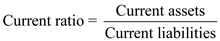Acid-test ratio:

Acid-test ratio is a liquidity ratio that excludes inventory and prepaid expenses being the non-liquid assets. It is also called quick ratio or liquid ratio. It is the most ideal ratio to determine the liquidity of the company. Ideal acid-test ratio is 1:1.

It is calculated as follows: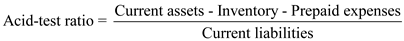Cash ratio:

Cash ratio is a type of liquidity ratio that determines the ability of the company to pay its current or short-term liabilities by the cash and cash equivalents of the company. Cash and cash equivalents refer to the most liquid assets of the company and includes assets such as marketable securities, commercial paper, treasury bills etc.

It is calculated as follows: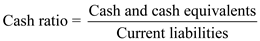Days’ sales in receivables:

It means estimation of days’ company would take to realize its debtors. It means time taken by customers to pay its credit to company. It is calculated by dividing accounts receivable by net sales multiply by 360. Net sales is sales after deducting discounts. It is calculated by following formula: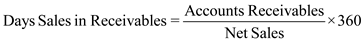a.

Working capital is calculated below:b.

The current ratio is calculated below:c.

The acid-test ratio is calculated below:d.

Cash ratio is calculated as below:e.

Days’ sales in receivables are calculated below:f.

Accounts receivable turnover in days is calculated below:g.

Days’ sales in inventory are calculated below:h.

Inventory turnover in days is calculated below:i.

Operating cycle is calculated below:Note: It is been assumed that there are 365 days in a year.